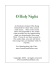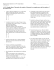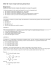# G.G.49: Chords 1: Investigate theorems regarding chords of a circle:... chords; the relative lengths of chords compared to their distance...

## Transcription

G.G.49: Chords 1: Investigate theorems regarding chords of a circle:... chords; the relative lengths of chords compared to their distance...
```Regents Exam Questions G.G.49: Chords 1
Name: ________________________
www.jmap.org
G.G.49: Chords 1: Investigate theorems regarding chords of a circle: perpendicular bisectors of
chords; the relative lengths of chords compared to their distance from the center
3 In the diagram below, circle O has a radius of 5,
and CE = 2. Diameter AC is perpendicular to
chord BD at E.
1 In circle O, diameter AB intersects chord CD at E.
If CE = ED, then ∠CEA is which type of angle?
1) straight
2) obtuse
3) acute
4) right
2 In the diagram below of circle O, radius OC is 5
cm. Chord AB is 8 cm and is perpendicular to OC
at point P.
What is the length of BD ?
1) 12
2) 10
3) 8
4) 4
What is the length of OP, in centimeters?
1) 8
2) 2
3) 3
4) 4
1
Regents Exam Questions G.G.49: Chords 1
Name: ________________________
www.jmap.org
4 In the diagram below of circle O, diameter AOB is
perpendicular to chord CD at point E, OA = 6, and
OE = 2.
6 In the diagram below, diameter AB bisects chord
CD at point E in circle F.
If AE = 2 and FB = 17 , then the length of CE is
1) 7
2) 8
3) 15
4) 16
What is the length of CE ?
1) 4 3
2) 2 3
3) 8 2
4) 4 2
7 In the diagram below of circle O, diameter AB is
perpendicular to chord CD at E. If AO = 10 and
BE = 4 , find the length of CE .
5 In circle O shown below, diameter DB is
perpendicular to chord AC at E.
If DB = 34 , AC = 30 , and DE > BE , what is the
length of BE ?
1) 8
2) 9
3) 16
4) 25
2
Regents Exam Questions G.G.49: Chords 1
Name: ________________________
www.jmap.org
8 In circle O shown below, chords AB and CD and
radius OA are drawn, such that AB ≅ CD,
OE ⊥ AB , OF ⊥ CD, OF = 16, CF = y + 10, and
CD = 4y − 20.
10 In the diagram below of circle O, diameter AB and
chord CD intersect at E.
If AB ⊥ CD, which statement is always true?
Determine the length of DF . Determine the length
of OA.
9 In circle R shown below, diameter DE is
perpendicular to chord ST at point L.
1)
AC ≅ BD
2)
BD ≅ DA
3)
4)
CB ≅ BD
11 In the diagram below, ABC is inscribed in circle
P. The distances from the center of circle P to each
side of the triangle are shown.
Which statement is not always true?
1) SL ≅ TL
2) RS = DR
3) RL ≅ LE
4) (DL)(LE) = (SL)(LT)
Which statement about the sides of the triangle is
true?
1) AB > AC > BC
2) AB < AC and AC > BC
3) AC > AB > BC
4) AC = AB and AB > BC
3
ID: A
G.G.49: Chords 1: Investigate theorems regarding chords of a circle: perpendicular bisectors of
chords; the relative lengths of chords compared to their distance from the center
1 ANS: 4
2 ANS: 3
REF: 081308ge
REF: 011112ge
3 ANS: 3
Because OC is a radius, its length is 5. Since CE = 2 OE = 3.
REF: fall0811ge
4 ANS: 4
62 − 22 =
32 =
16
2 =4
2
REF: 081124ge
5 ANS: 2
17 2 − 15 2 = 8. 17 − 8 = 9
REF: 061221ge
6 ANS: 2
17 2 − 15 2 =
289 − 225 =
64 = 8
REF: 011424ge
7 ANS:
EO = 6 . CE =
10 2 − 6 2 = 8
REF: 011234ge
1
EDO is a 3-4-5 triangle. If ED = 4 , BD = 8 .
ID: A
8 ANS:
2(y + 10) = 4y − 20. DF = y + 10 = 20 + 10 = 30. OA = OD =
16 2 + 30 2 = 34
2y + 20 = 4y − 20
40 = 2y
20 = y
REF: 061336ge
9 ANS: 3
REF: 011322ge
10 ANS: 4
REF: 081403ge
11 ANS: 1
The closer a chord is to the center of a circle, the longer the chord.
REF: 011005ge
2
```

### Root Position 3-Note Chords www.JasonWerkema.com (for 6-string Bass)### square, circle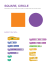### Lesson BR6a - Where Did You Sleep Last Night www.brightonguitarlessons.co.uk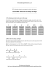### 12-2 Practice Chords and Arcs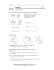### Tips and Tricks for Sight-Reading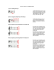### fire stone home products outback chair company laneventure gloster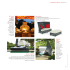### Name: __________________________________________________________________ Period: ___________________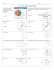### CONTENTS : Chord Book 1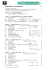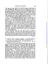### O Holy Night